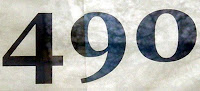## Tuesday, August 31, 2010

### 490

490 = 2 x 5 x 72.

490 has a representation as a sum of two squares: 490 = 72 + 212.

490 is the number of integer partitions of 19.

490 is the sum of six positive fifth powers: 490 = 15 + 15 + 15 + 15 + 35 + 35.The USS Leader (MSO 490) was a minesweeper launched in 1954.